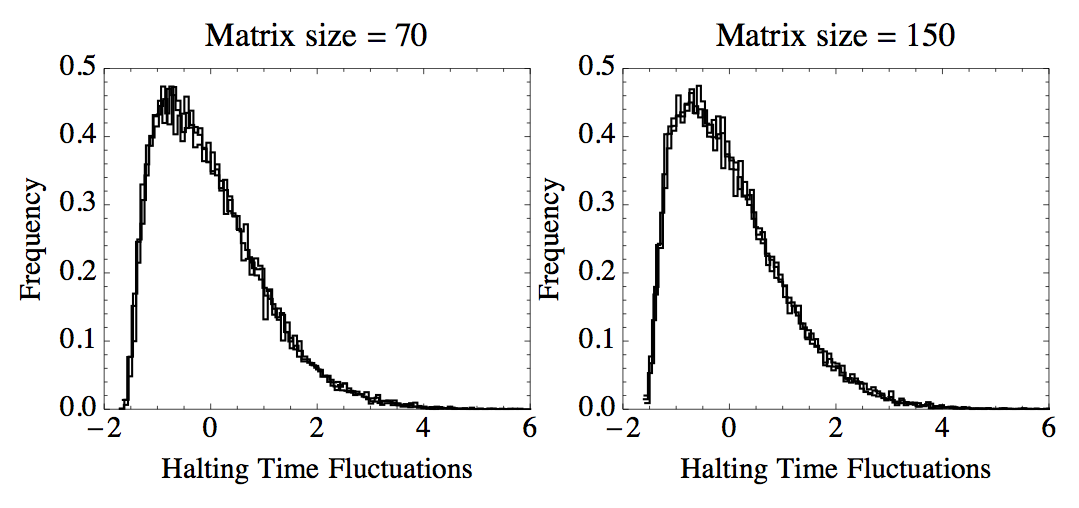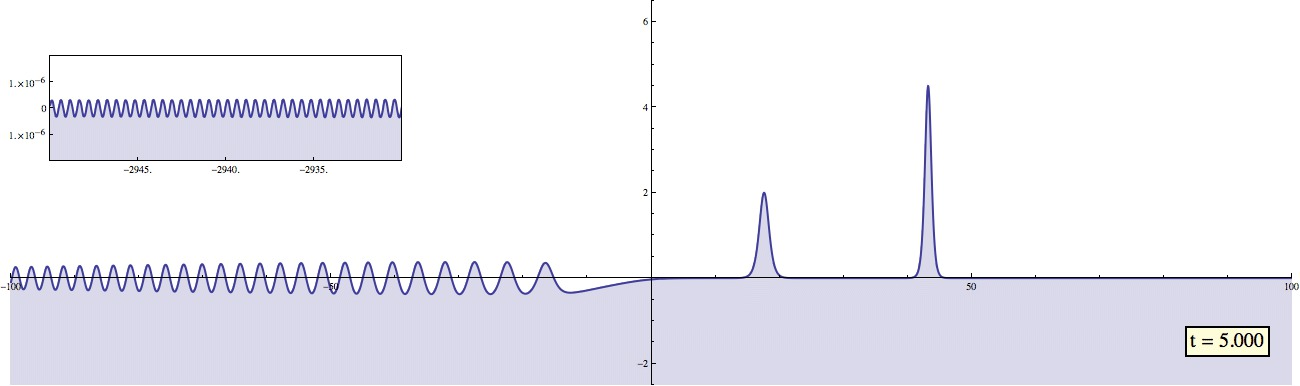### NumericalUniversality

NumericalUniversality is a Mathematica library that contains a database, algorithms and random matrix distributions for examining two-component universality in the halting times for these algorithms.

NumericalUniversality Repository

A full wiki article is available above to describe the use of the package.Two-component universality in the QR eigenvalue algorithm

### ISTPackage

ISTPackage is a Mathematica package that contains various routines for effectively evaluating the inverse scattering transform. Thus far it has been implemented for the Korteweg-de Vries (KdV) equation, the focusing and defocusing modified KdV equations and the focusing and defocusing nonlinear Schrödinger (NLS) equations. It also contains a routine for the linear Schrödinger equation. The code can be found on bitbucket:

ISTPackage Repository

The package allows for the computation of highly oscillatory solutions. The animation below includes a picture-in-picture evolution of a solution of the KdV equation. The animation tracks both the dispersive tail moving to the left and the solitons moving to the right.A highly oscillatory solution of the KdV equation

### Hill's Method

The following is a Python-based implementation of Hill's method. The software includes a GTK-based GUI. This project is no longer being maintained.

Hill's Method in Python